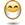# Sortino Ratio - Downside Deviation versus Semi-Deviation

## Excel Help for Sortino Ratio - Downside Deviation Versus Semi-deviation in Excel Portfolio Optimization TemplateHello,I am trying to understand the differences between the downside deviation and semi-deviation calculations for the two sortino ratio options for the portfolio optimization model.The semi-deviation model only calculates volatility below a target return level, so theoretically, if target return and RfR are 0%, then the raw negative asset returns shown would be used to calculate the downside deviation. However, for the downside deviation option, the model (help box) states that "only volatility below the average is used" - what average is the model referring to? The overall return? The target return?As well, how do you actually calculate the downside deviation or standard deviation more generally? There are multiple methods of calculating sortino ratios and I am unable to match what the optimization model produces with my own calculations of sortino/sharpe ratios (the total returns match, but the standard deviations are slightly off).Many thanks in advance. Posted by A.Yu on 21 Jul 2015Rate this: (3/5 from 1 vote) The difference between downside and semi-deviation is only that downside uses the average return as a target while semi uses the target rate specified.Both calculations account for periods during the time series of data when the return is below this target and the variance thereof to calculate deviation.  This differs from standard deviation which looks at all variance of returns both up and down.The only circumstance where downside or semi deviation can be zero is when all periods in the time frame have returns greater than the target rate i.e. a continuous uptrend. Excel Business Forums Administrator Posted by Excel Helper on 21 Jul 2015Rate this: (3/5 from 1 vote) Thank you for help - when you mentioned average return, where is that figure derived from? The benchmark? Or the average of each asset? Posted by A.Yu on 21 Jul 2015Rate this: (4/5 from 2 votes) The average return for downside deviation is the average of returns for all periods. If the deviation is for the asset then it is the asset returns and, if the entire portfolio, then it is the weighted average returns of all assets using the portfolio weightings (existing or optimized). Excel Business Forums Administrator Posted by Excel Helper on 21 Jul 2015